# MCAT Physics Practice Test 4: Fluids

### Test Information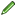15 questions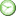minutes

Take more free MCAT physics practice tests available from maintests.com.

1. Objects A and B are submerged at a depth of 1 m in a liquid with a specific gravity of 0.877. Given that the density of object B is one-third that of object A and that the gauge pressure of object A is 3 atm, what is the gauge pressure of object B? (Note: Assume atmospheric pressure is 1 atm and)

• A. 1 atm
• B. 2 atm
• C. 3 atm
• D. 9 atm

2. An anchor made of iron weighs 833 N on the deck of a ship. If the anchor is now suspended in seawater by a massless chain, what is the tension in the chain? (Note: The density of iron is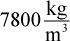and the density of seawater is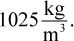)

• A. 100 N
• B. 724 N
• C. 833 N
• D. 957 N

3. Two wooden balls of equal volume but different density are held beneath the surface of a container of water. Ball A has a density of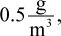and ball B has a density of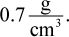When the balls are released, they will accelerate upward to the surface. What is the relationship between the acceleration of ball A and that of ball B?

• A. Ball A has the greater acceleration.
• B. Ball B has the greater acceleration.
• C. Balls A and B have the same acceleration.
• D. It cannot be determined from information given.

4. Water flows from a pipe of diameter 0.15 m into one of diameter 0.2 m. If the speed in the 0.15 m pipe is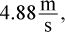, what is the speed in the 0.2 m pipe?

• A.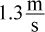• B.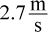• C.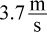• D.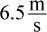5. A hydraulic lever is used to lift a heavy hospital bed, requiring an amount of work W. When the same bed with a patient is lifted, the work required is doubled. How can the cross-sectional area of the platform on which the bed is lifted be changed so that the pressure on the hydraulic lever remains constant?

• A. The cross-sectional area must be doubled.
• B. The cross-sectional area must be halved.
• C. The cross-sectional area must be divided by four.
• D. The cross-sectional area must remain constant.

6. The figure shown represents a section through a horizontal pipe of varying diameters into which four open vertical pipes connect. If water is allowed to flow through the pipe in the direction indicated, in which of the vertical pipes will the water level be lowest?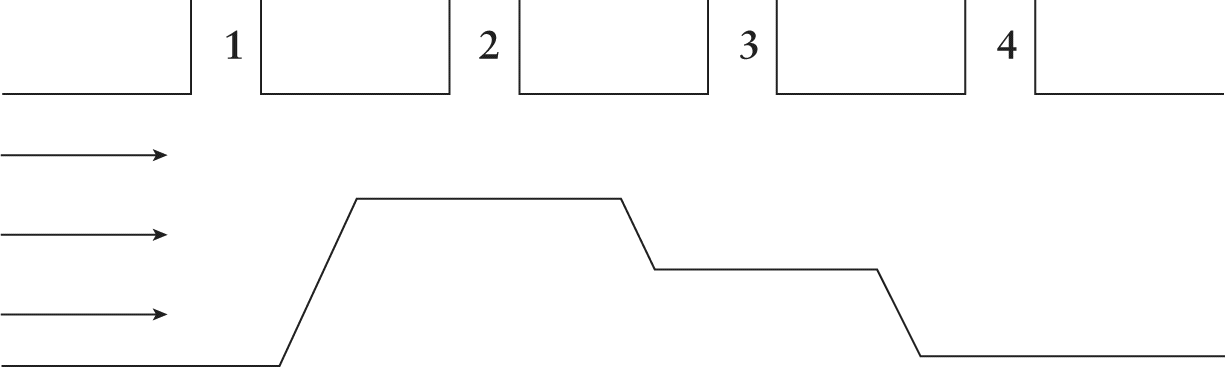• A. Pipe 1
• B. Pipe 2
• C. Pipe 3
• D. Pipe 4

7. The speed of blood in the aorta is much higher than the speed of blood through a capillary bed. How can this fact be explained using the continuity equation, assuming that we are interested in average flow and that there is no net fluid loss?

• A. The aorta is located higher than the capillary bed.
• B. The pressure in the aorta is the same as the pressure in the capillary bed.
• C. The cross-sectional area of all the capillaries added together is much greater than the cross-sectional area of the aorta.
• D. The cross-sectional area of a capillary is much smaller than the cross-sectional area of the aorta.

8. Which of the following data sets is sufficient to determine the linear speed through an area of a rigid pipe?

• A. The cross sectional area in another segment of pipe and the cross sectional area in the region of interest
• B. The Reynolds number, viscosity of the fluid, density, and diameter of the pipe
• C. The radius of the pipe, pressure gradient, viscosity, and length of the pipe
• D. The absolute pressure and density

9. A large cylinder is filled with equal volumes of two immiscible fluids. A balloon is submerged in the first fluid; the gauge pressure in the balloon at the deepest point in the first fluid is found to be 3 atm. Next, the balloon is lowered all the way to the bottom of the second fluid, where the hydrostatic pressure in the balloon reads 8 atm. What is the ratio of the gauge pressure accounted for by the first fluid to the gauge pressure accounted for by the second fluid?

• A. 1:03
• B. 3:04
• C. 3:05
• D. 3:08

10. An hydraulic system is designed to allow water levels to change depending on a force applied at the top of the tank as shown. If a force, F1, of 4 N is applied to a square, flexible cover where A1 = 16, and the area A2 = 64, what force must be applied to A2 to keep the water levels from changing?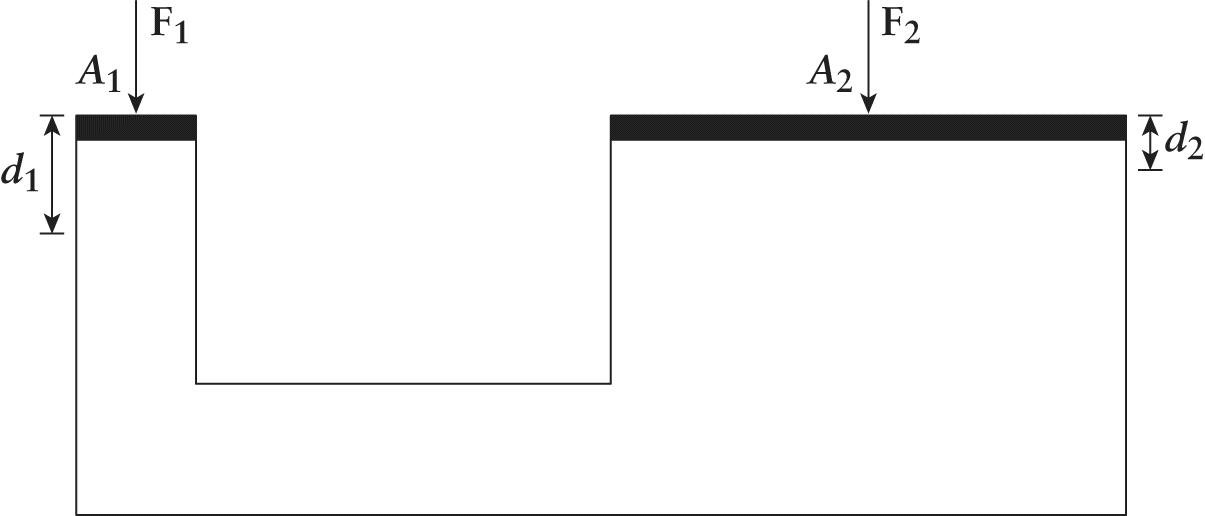• A. 4 N
• B. 16 N
• C. 32 N
• D. No force needs to be applied.

11. Balls A and B of equal mass are floating in a swimming pool, as shown below. Which will produce a greater buoyant force?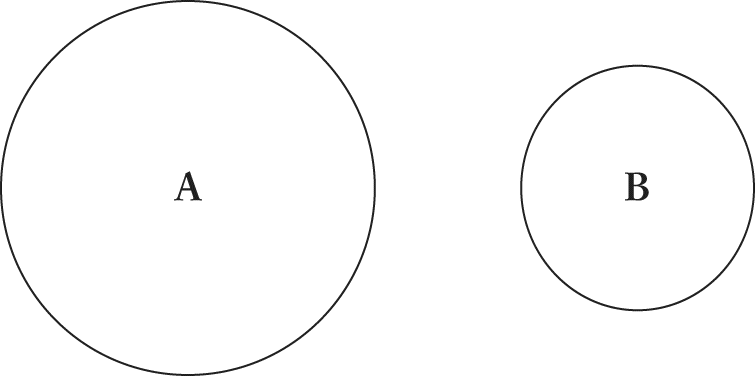• A. Ball A
• B. Ball B
• C. The forces will be equal.
• D. It is impossible to know without knowing the volume of each ball.

12. Bernoulli's equation is the reason for the upward force that permits airplane flight. Which statement best summarizes the equation's relationship to flight?

• A. The speed of airflow is equal on the top and bottom of a wing, resulting in nonturbulent flight.
• B. The speed of airflow is greater over the curved top of the wing, resulting in less pressure on the top of the wing and the production of a net upward force on the wing, in turn resulting in flight.
• C. The speed of airflow on the flat bottom of the wing is greater than over the curved top of the wing, resulting in more pressure below the wing and the production of a net upward force on the wing, in turn resulting in flight.
• D. The weight of the wing is directly proportional to the weight of the air it displaces.

13. A low-pressure weather system can decrease the atmospheric pressure from 1 atm to 0.99 atm. By what percent will this decrease the force on a rectangular window from the outside? (Note: Assume the window is 6 m by 3 m and the glass is 3 cm thick.)

• A. 1%
• B. 10%
• C.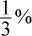• D. 30%

14. Two fluids, A and B, have densities of x and 2x, respectively. They are tested independently to assess absolute pressure at varying depths. At what depths will the pressure below the surface of these two fluids be equal?

• A. Whenever the depth of fluid A is one-half that of fluid B
• B. Whenever the depth of fluid A equals that of fluid B
• C. Whenever the depth of fluid A is 2 times that of fluid B
• D. Whenever the depth of fluid A is 4 times that of fluid B

15. A water tower operator is interested in increasing the pressure of a column of water that is applied to a piston. She hopes that increasing the pressure will increase the force being applied to the piston. The only way to increase the pressure is to alter the speed of the water as it flows through the pipe to the piston. How should the speed of the water be changed to increase the pressure and force?

• A. Increase the speed
• B. Decrease the speed
• C. Release water intermittently against the pipe
• D. The speed of water will not change pressure at the piston.

﻿